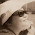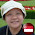## Wednesday, 17 February 2016

### Algebra... Which is larger? (II)

Jennifer says, "5x2  is always smaller than  (-5x)2. "

Indicate any assumptions made.

This question is compulsory.

1.no. This is because 5x to the power of two is 25x but -5x to the power of two is also 25x(positive) because the product of negative numbers and negative numbers will give you positive numbers

1.add on:so they are equal

2.This comment has been removed by the author.

2.No. As (-5x) times 5x will make it positive and the answer will be 25x . 5x times 5x will make it 25x too so both are the same.

3.They are both eaqual as a negative times negative is positive, and both the numbers are 25x in the end

1.4.This comment has been removed by the author.

5.No as
5x X 5x = 25x
-5x X -5x = 25x
So (5x)^2 = (-5x)^2

6.No. They are the same as 5x times itself is 25x and -5x times itself (-X-=+) is also 25x

7.No,as 5x to the power of two is 25 and (-5x) to the power of two is also 25 because negative times negative gives a positive number.Therefore they are equal.

8.No,as 5x to the power of two is 25 and (-5x) to the power of two is also 25 because negative times negative gives a positive number.Therefore they are equal.

9.No, they can be equal. negative and negative equal positive, so they will be equal as 25x

10.No.As negative and negative times together gives a positive,so both are still equivalent to 25x

11.No.They are equal since 2 negative numbers multiple to have a positive number(in this case the power of 2 which is even).Both will be exactly the same

12.No.A negative number squared gives a positive number.If they are of the same magnitude,they will be equal which is 25x

13.This comment has been removed by the author.

14.No. They are equal as negative times negative will give you positive(-5x)(-5x)=25x. Positive times positive will give you positive(5x)(5x)=25.

15.No. Negative times negative equals positive so they are equal.

16.no. Because negative X negative = positive, which will be the same as the first one.

17.no. Because negative X negative = positive, which will be the same as the first one.

18.Both of the answers are the same as '-' times '-' gives a positive, which results in the same answer as both statements have nothing else different other than the negative sign.

19.No. 'x' might be 0 (assuming) so 5 x 0=0 and (-5x)^(2) also equals to 0. Also, x might be 2 so 5x times 2 (assuming) equals to 10x and because (-5x)^(2) is also can be expressed as -5x times -5x equals 25x as there are double negatives, the final answer will be in positive.

20.Good. You highlighted the possibility of having both terms being equal by testing it with ZERO.
This is one possible scenario.

21.Note: only a member of this blog may post a comment.